# Train a Twin Neural Network to Compare Images

This example shows how to train a twin neural network with shared weights to identify similar images of handwritten characters.

A twin neural network is a type of deep learning network that uses two or more identical subnetworks that have the same architecture and share the same parameters and weights. Twin networks are typically used in tasks that involve finding the relationship between two comparable things. Some common applications for twin networks include facial recognition, signature verification , or paraphrase identification . Twin networks perform well in these tasks because their shared weights mean there are fewer parameters to learn during training and they can produce good results with a relatively small amount of training data.

Twin networks are particularly useful in cases where there are large numbers of classes with small numbers of observations of each. In such cases, there is not enough data to train a deep convolutional neural network to classify images into these classes. Instead, the twin network can determine if two images are in the same class.

This example use the Omniglot dataset  to train a twin network to compare images of handwritten characters . The Omniglot dataset contains character sets for 50 alphabets, divided into 30 used for training and 20 for testing. Each alphabet contains a number of characters from 14 for Ojibwe (Canadian Aboriginal syllabics) to 55 for Tifinagh. Finally, each character has 20 handwritten observations. This example trains a network to identify whether two handwritten observations are different instances of the same character.

You can also use twin networks to identify similar images using dimensionality reduction. For an example, see Train a Twin Network for Dimensionality Reduction.

### Load and Preprocess Training Data

```url = "https://github.com/brendenlake/omniglot/raw/master/python/images_background.zip"; downloadFolder = tempdir; filename = fullfile(downloadFolder,"images_background.zip"); dataFolderTrain = fullfile(downloadFolder,"images_background"); if ~exist(dataFolderTrain,"dir") disp("Downloading Omniglot training data (4.5 MB)...") websave(filename,url); unzip(filename,downloadFolder); end```
```Downloading Omniglot training data (4.5 MB)... ```
`disp("Training data downloaded.")`
```Training data downloaded. ```

Load the training data as a image datastore using the `imageDatastore` function. Specify the labels manually by extracting the labels from the file names and setting the `Labels` property.

```imdsTrain = imageDatastore(dataFolderTrain, ... IncludeSubfolders=true, ... LabelSource="none"); files = imdsTrain.Files; parts = split(files,filesep); labels = join(parts(:,(end-2):(end-1)),"-"); imdsTrain.Labels = categorical(labels);```

The Omniglot training dataset consists of black and white handwritten characters from 30 alphabets, with 20 observations of each character. The images are of size 105-by-105-by-1, and the values of each pixel are between `0` and `1`.

Display a random selection of the images.

```idx = randperm(numel(imdsTrain.Files),8); for i = 1:numel(idx) subplot(4,2,i) imshow(readimage(imdsTrain,idx(i))) title(imdsTrain.Labels(idx(i)),Interpreter="none"); end```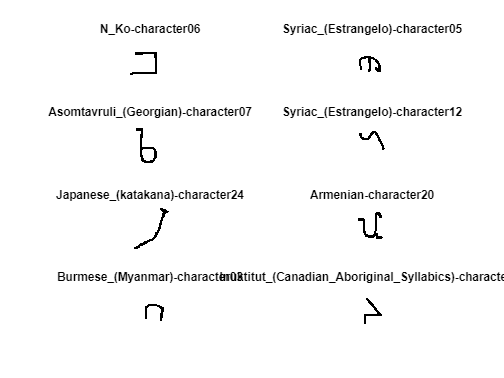### Create Pairs of Similar and Dissimilar Images

To train the network, the data must be grouped into pairs of images that are either similar or dissimilar. Here, similar images are different handwritten instances of the same character, which have the same label, while dissimilar images of different characters have different labels. The function `getTwinBatch` (defined in the Supporting Functions section of this example) creates randomized pairs of similar or dissimilar images, `pairImage1 `and `pairImage2`. The function also returns the label `pairLabel`, which identifies if the pair of images is similar or dissimilar to each other. Similar pairs of images have `pairLabel = 1`, while dissimilar pairs have `pairLabel = 0`.

As an example, create a small representative set of five pairs of images

```batchSize = 10; [pairImage1,pairImage2,pairLabel] = getTwinBatch(imdsTrain,batchSize);```

Display the generated pairs of images.

```for i = 1:batchSize if pairLabel(i) == 1 s = "similar"; else s = "dissimilar"; end subplot(2,5,i) imshow([pairImage1(:,:,:,i) pairImage2(:,:,:,i)]); title(s) end```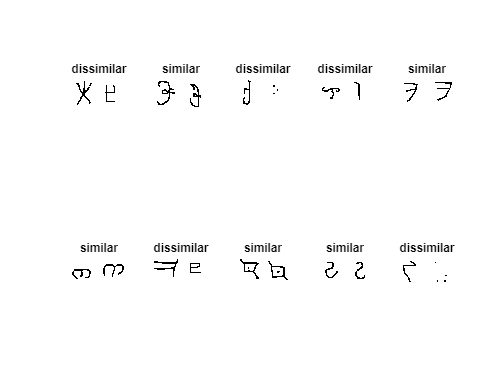In this example, a new batch of 180 paired images is created for each iteration of the training loop. This ensures that the network is trained on a large number of random pairs of images with approximately equal proportions of similar and dissimilar pairs.

### Define Network Architecture

The twin network architecture is illustrated in the following diagram.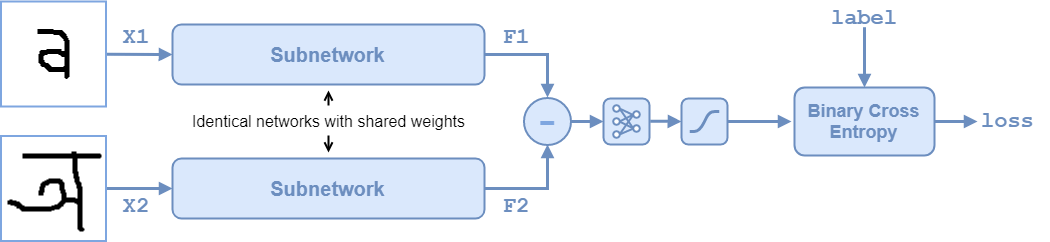To compare two images, each image is passed through one of two identical subnetworks that share weights. The subnetworks convert each 105-by-105-by-1 image to a 4096-dimensional feature vector. Images of the same class have similar 4096-dimensional representations. The output feature vectors from each subnetwork are combined through subtraction and the result is passed through a `fullyconnect` operation with a single output. A `sigmoid` operation converts this value to a probability between `0` and `1`, indicating the network prediction of whether the images are similar or dissimilar. The binary cross-entropy loss between the network prediction and the true label is used to update the network during training.

In this example, the two identical subnetworks are defined as a `dlnetwork` object. The final `fullyconnect` and `sigmoid` operations are performed as functional operations on the subnetwork outputs.

Create the subnetwork as a series of layers that accepts 105-by-105-by-1 images and outputs a feature vector of size 4096.

For the `convolution2dLayer` objects, use the narrow normal distribution to initialize the weights and bias.

For the `maxPooling2dLayer` objects, set the stride to `2`.

For the final `fullyConnectedLayer` object, specify an output size of 4096 and use the narrow normal distribution to initialize the weights and bias.

```layers = [ imageInputLayer([105 105 1],Normalization="none") convolution2dLayer(10,64,WeightsInitializer="narrow-normal",BiasInitializer="narrow-normal") reluLayer maxPooling2dLayer(2,Stride=2) convolution2dLayer(7,128,WeightsInitializer="narrow-normal",BiasInitializer="narrow-normal") reluLayer maxPooling2dLayer(2,Stride=2) convolution2dLayer(4,128,WeightsInitializer="narrow-normal",BiasInitializer="narrow-normal") reluLayer maxPooling2dLayer(2,Stride=2) convolution2dLayer(5,256,WeightsInitializer="narrow-normal",BiasInitializer="narrow-normal") reluLayer fullyConnectedLayer(4096,WeightsInitializer="narrow-normal",BiasInitializer="narrow-normal")]; ```

To train the network with a custom training loop and enable automatic differentiation, convert the layer graph to a `dlnetwork` object.

`net = dlnetwork(layers);`

Create the weights for the final `fullyconnect` operation. Initialize the weights by sampling a random selection from a narrow normal distribution with standard deviation of 0.01.

```fcWeights = dlarray(0.01*randn(1,4096)); fcBias = dlarray(0.01*randn(1,1)); fcParams = struct(... "FcWeights",fcWeights,... "FcBias",fcBias);```

To use the network, create the function `forwardTwin` (defined in the Supporting Functions section of this example) that defines how the two subnetworks and the subtraction, `fullyconnect`, and `sigmoid` operations are combined. The function `forwardTwin` accepts the network, the structure containing the parameters for the `fullyconnect` operation, and two training images. The `forwardTwin` function outputs a prediction about the similarity of the two images.

### Define Model Loss Function

Create the function `modelLoss` (defined in the Supporting Functions section of this example). The `modelLoss` function takes the subnetwork `net`, the parameter structure for the `fullyconnect` operation, and a mini-batch of input data `X1` and `X2` with their labels `pairLabels`. The function returns the loss values and the gradients of the loss with respect to the learnable parameters of the network.

The objective of the twin network is to discriminate between the two inputs `X1` and `X2`. The output of the network is a probability between `0` and `1`, where a value closer to `0` indicates a prediction that the images are dissimilar, and a value closer to `1` that the images are similar. The loss is given by the binary cross-entropy between the predicted score and the true label value:

`$\mathrm{loss}=-\mathrm{tlog}\left(\mathit{y}\right)-\left(1-\mathit{t}\right)\mathrm{log}\left(1-\mathit{y}\right),$`

where the true label $\mathit{t}$ can be 0 or 1 and $\mathit{y}$ is the predicted label.

### Specify Training Options

Specify the options to use during training. Train for 10000 iterations.

```numIterations = 10000; miniBatchSize = 180;```

Specify the options for ADAM optimization:

• Set the learning rate to `0.00006`.

• Set the gradient decay factor to `0.9` and the squared gradient decay factor to `0.99`.

```learningRate = 6e-5; gradDecay = 0.9; gradDecaySq = 0.99;```

Train on a GPU, if one is available. Using a GPU requires Parallel Computing Toolbox™ and a supported GPU device. For information on supported devices, see GPU Computing Requirements (Parallel Computing Toolbox). To automatically detect if you have a GPU available and place the relevant data on the GPU, set the value of `executionEnvironment` to `"auto"`. If you do not have a GPU, or do not want to use one for training, set the value of `executionEnvironment` to `"cpu"`. To ensure you use a GPU for training, set the value of `executionEnvironment` to `"gpu"`.

`executionEnvironment = "auto";`

Check whether a GPU is available for training.

```if canUseGPU gpu = gpuDevice; disp(gpu.Name + " GPU detected and available for training.") end```
```NVIDIA RTX A5000 GPU detected and available for training. ```

### Train Model

Initialize the parameters for the ADAM solver.

```trailingAvgSubnet = []; trailingAvgSqSubnet = []; trailingAvgParams = []; trailingAvgSqParams = [];```

Initialize the `TrainingProgressMonitor` object. Because the timer starts when you create the monitor object, make sure that you create the object close to the training loop.

```monitor = trainingProgressMonitor(Metrics="Loss",XLabel="Iteration",Info="ExecutionEnvironment"); if canUseGPU updateInfo(monitor,ExecutionEnvironment=gpu.Name + " GPU") else updateInfo(monitor,ExecutionEnvironment="CPU") end```

Train the model using a custom training loop. Loop over the training data and update the network parameters at each iteration.

For each iteration:

• Extract a batch of image pairs and labels using the `getTwinBatch` function defined in the section Create Batches of Image Pairs.

• Convert the data to `dlarray` objects specify the dimension labels `"SSCB"` (spatial, spatial, channel, batch) for the image data and `"CB"` (channel, batch) for the labels.

• For GPU training, convert the data to `gpuArray` objects.

• Evaluate the model loss and gradients using `dlfeval` and the `modelLoss` function.

• Update the network parameters using the `adamupdate` function.

• Record the training loss in the training progress monitor.

• Stop if the `Stop` property is `true`. The `Stop` property value of the `TrainingProgressMonitor` object changes to `true` when you click the `Stop` button.

```start = tic; iteration = 0; % Loop over mini-batches. while iteration < numIterations && ~monitor.Stop iteration = iteration + 1; % Extract mini-batch of image pairs and pair labels [X1,X2,pairLabels] = getTwinBatch(imdsTrain,miniBatchSize); % Convert mini-batch of data to dlarray. Specify the dimension labels % "SSCB" (spatial, spatial, channel, batch) for image data X1 = dlarray(X1,"SSCB"); X2 = dlarray(X2,"SSCB"); % If training on a GPU, then convert data to gpuArray. if (executionEnvironment == "auto" && canUseGPU) || executionEnvironment == "gpu" X1 = gpuArray(X1); X2 = gpuArray(X2); end % Evaluate the model loss and gradients using dlfeval and the modelLoss % function listed at the end of the example. [loss,gradientsSubnet,gradientsParams] = dlfeval(@modelLoss,net,fcParams,X1,X2,pairLabels); % Update the twin subnetwork parameters. [net,trailingAvgSubnet,trailingAvgSqSubnet] = adamupdate(net,gradientsSubnet, ... trailingAvgSubnet,trailingAvgSqSubnet,iteration,learningRate,gradDecay,gradDecaySq); % Update the fullyconnect parameters. [fcParams,trailingAvgParams,trailingAvgSqParams] = adamupdate(fcParams,gradientsParams, ... trailingAvgParams,trailingAvgSqParams,iteration,learningRate,gradDecay,gradDecaySq); % Update the training progress monitor. recordMetrics(monitor,iteration,Loss=loss); monitor.Progress = 100 * iteration/numIterations; end```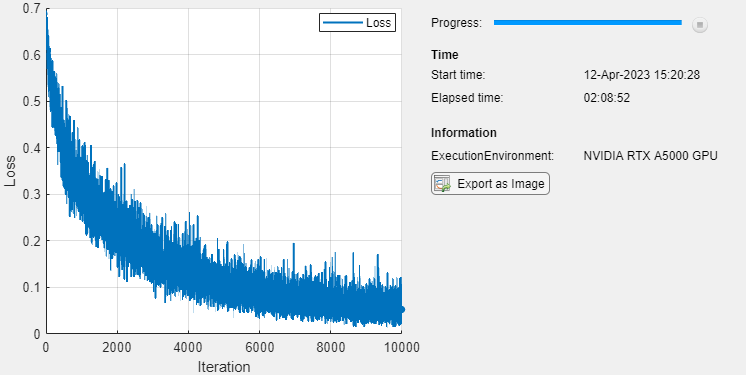### Evaluate the Accuracy of the Network

```url = "https://github.com/brendenlake/omniglot/raw/master/python/images_evaluation.zip"; downloadFolder = tempdir; filename = fullfile(downloadFolder,"images_evaluation.zip"); dataFolderTest = fullfile(downloadFolder,"images_evaluation"); if ~exist(dataFolderTest,"dir") disp("Downloading Omniglot test data (3.2 MB)...") websave(filename,url); unzip(filename,downloadFolder); end```
```Downloading Omniglot test data (3.2 MB)... ```
`disp("Test data downloaded.")`
```Test data downloaded. ```

Load the test data as a image datastore using the `imageDatastore` function. Specify the labels manually by extracting the labels from the file names and setting the `Labels` property.

```imdsTest = imageDatastore(dataFolderTest, ... IncludeSubfolders=true, ... LabelSource="none"); files = imdsTest.Files; parts = split(files,filesep); labels = join(parts(:,(end-2):(end-1)),"_"); imdsTest.Labels = categorical(labels);```

The test dataset contains 20 alphabets that are different to those that the network was trained on. In total, there 659 different classes in the test dataset.

`numClasses = numel(unique(imdsTest.Labels))`
```numClasses = 659 ```

To calculate the accuracy of the network, create a set of five random mini-batches of test pairs. Use the `predictTwin` function (defined in the Supporting Functions section of this example) to evaluate the network predictions and calculate the average accuracy over the mini-batches.

```accuracy = zeros(1,5); accuracyBatchSize = 150; for i = 1:5 % Extract mini-batch of image pairs and pair labels [X1,X2,pairLabelsAcc] = getTwinBatch(imdsTest,accuracyBatchSize); % Convert mini-batch of data to dlarray. Specify the dimension labels % "SSCB" (spatial, spatial, channel, batch) for image data. X1 = dlarray(X1,"SSCB"); X2 = dlarray(X2,"SSCB"); % If using a GPU, then convert data to gpuArray. if (executionEnvironment == "auto" && canUseGPU) || executionEnvironment == "gpu" X1 = gpuArray(X1); X2 = gpuArray(X2); end % Evaluate predictions using trained network Y = predictTwin(net,fcParams,X1,X2); % Convert predictions to binary 0 or 1 Y = gather(extractdata(Y)); Y = round(Y); % Compute average accuracy for the minibatch accuracy(i) = sum(Y == pairLabelsAcc)/accuracyBatchSize; end```

Compute accuracy over all minibatches

`averageAccuracy = mean(accuracy)*100`
```averageAccuracy = 89.2000 ```

### Display a Test Set of Images with Predictions

To visually check if the network correctly identifies similar and dissimilar pairs, create a small batch of image pairs to test. Use the `predictTwin` function to get the prediction for each test pair. Display the pair of images with the prediction, the probability score, and a label indicating whether the prediction was correct or incorrect.

```testBatchSize = 10; [XTest1,XTest2,pairLabelsTest] = getTwinBatch(imdsTest,testBatchSize);```

Convert the test batch of data to `dlarray`. Specify the dimension labels `"SSCB"` (spatial, spatial, channel, batch) for image data.

```XTest1 = dlarray(XTest1,"SSCB"); XTest2 = dlarray(XTest2,"SSCB");```

If using a GPU, then convert the data to `gpuArray`.

```if (executionEnvironment == "auto" && canUseGPU) || executionEnvironment == "gpu" XTest1 = gpuArray(XTest1); XTest2 = gpuArray(XTest2); end```

Calculate the predicted probability.

```YScore = predictTwin(net,fcParams,XTest1,XTest2); YScore = gather(extractdata(YScore));```

Convert the predictions to binary 0 or 1.

`YPred = round(YScore);`

Extract the data for plotting.

```XTest1 = extractdata(XTest1); XTest2 = extractdata(XTest2);```

Plot images with predicted label and predicted score.

```f = figure; tiledlayout(2,5); f.Position(3) = 2*f.Position(3); predLabels = categorical(YPred,[0 1],["dissimilar" "similar"]); targetLabels = categorical(pairLabelsTest,[0 1],["dissimilar","similar"]); for i = 1:numel(pairLabelsTest) nexttile imshow([XTest1(:,:,:,i) XTest2(:,:,:,i)]); title( ... "Target: " + string(targetLabels(i)) + newline + ... "Predicted: " + string(predLabels(i)) + newline + ... "Score: " + YScore(i)) end```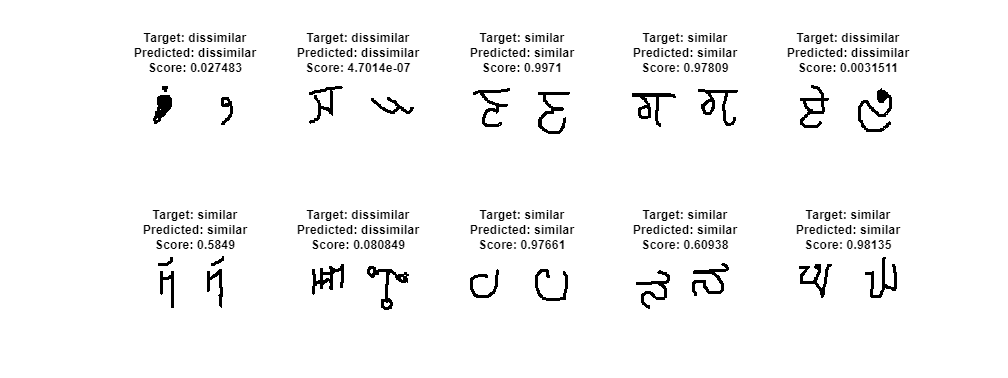The network is able to compare the test images to determine their similarity, even though none of these images were in the training dataset.

### Supporting Functions

#### Model Functions for Training and Prediction

The function `forwardTwin` is used during network training. The function defines how the subnetworks and the `fullyconnect` and `sigmoid` operations combine to form the complete twin network. The `forwardTwin` function accepts the network structure and two training images and outputs a prediction about the similarity of the two images. Within this example, the function `forwardTwin` is introduced in the section Define Network Architecture.

```function Y = forwardTwin(net,fcParams,X1,X2) % forwardTwin accepts the network and pair of training images, and % returns a prediction of the probability of the pair being similar (closer % to 1) or dissimilar (closer to 0). Use forwardTwin during training. % Pass the first image through the twin subnetwork Y1 = forward(net,X1); Y1 = sigmoid(Y1); % Pass the second image through the twin subnetwork Y2 = forward(net,X2); Y2 = sigmoid(Y2); % Subtract the feature vectors Y = abs(Y1 - Y2); % Pass the result through a fullyconnect operation Y = fullyconnect(Y,fcParams.FcWeights,fcParams.FcBias); % Convert to probability between 0 and 1. Y = sigmoid(Y); end```

The function `predictTwin` uses the trained network to make predictions about the similarity of two images. The function is similar to the function `forwardTwin`, defined previously. However, `predictTwin` uses the `predict` function with the network instead of the `forward` function, because some deep learning layers behave differently during training and prediction. Within this example, the function `predictTwin` is introduced in the section Evaluate the Accuracy of the Network.

```function Y = predictTwin(net,fcParams,X1,X2) % predictTwin accepts the network and pair of images, and returns a % prediction of the probability of the pair being similar (closer to 1) or % dissimilar (closer to 0). Use predictTwin during prediction. % Pass the first image through the twin subnetwork. Y1 = predict(net,X1); Y1 = sigmoid(Y1); % Pass the second image through the twin subnetwork. Y2 = predict(net,X2); Y2 = sigmoid(Y2); % Subtract the feature vectors. Y = abs(Y1 - Y2); % Pass result through a fullyconnect operation. Y = fullyconnect(Y,fcParams.FcWeights,fcParams.FcBias); % Convert to probability between 0 and 1. Y = sigmoid(Y); end```

#### Model Loss Function

The function `modelLoss` takes the twin `dlnetwork` object `net`, a pair of mini-batch input data `X1` and `X2`, and the label indicating whether they are similar or dissimilar. The function returns the binary cross-entropy loss between the prediction and the ground truth and the gradients of the loss with respect to the learnable parameters in the network. Within this example, the function `modelLoss` is introduced in the section Define Model Loss Function.

```function [loss,gradientsSubnet,gradientsParams] = modelLoss(net,fcParams,X1,X2,pairLabels) % Pass the image pair through the network. Y = forwardTwin(net,fcParams,X1,X2); % Calculate binary cross-entropy loss. loss = crossentropy(Y,pairLabels,ClassificationMode="multilabel"); % Calculate gradients of the loss with respect to the network learnable % parameters. [gradientsSubnet,gradientsParams] = dlgradient(loss,net.Learnables,fcParams); end```

#### Create Batches of Image Pairs

The following functions create randomized pairs of images that are similar or dissimilar, based on their labels. Within this example, the function `getTwinBatch` is introduced in the section Create Pairs of Similar and Dissimilar Images.

#### Get Twin Batch Function

The `getTwinBatch` returns a randomly selected batch of paired images. On average, this function produces a balanced set of similar and dissimilar pairs.

```function [X1,X2,pairLabels] = getTwinBatch(imds,miniBatchSize) % Initialize the output. pairLabels = zeros(1,miniBatchSize); imgSize = size(readimage(imds,1)); X1 = zeros([imgSize 1 miniBatchSize],"single"); X2 = zeros([imgSize 1 miniBatchSize],"single"); % Create a batch containing similar and dissimilar pairs of images. for i = 1:miniBatchSize choice = rand(1); % Randomly select a similar or dissimilar pair of images. if choice < 0.5 [pairIdx1,pairIdx2,pairLabels(i)] = getSimilarPair(imds.Labels); else [pairIdx1,pairIdx2,pairLabels(i)] = getDissimilarPair(imds.Labels); end X1(:,:,:,i) = imds.readimage(pairIdx1); X2(:,:,:,i) = imds.readimage(pairIdx2); end end```

#### Get Similar Pair Function

The `getSimilarPair` function returns a random pair of indices for images that are in the same class and the similar pair label equals 1.

```function [pairIdx1,pairIdx2,pairLabel] = getSimilarPair(classLabel) % Find all unique classes. classes = unique(classLabel); % Choose a class randomly which will be used to get a similar pair. classChoice = randi(numel(classes)); % Find the indices of all the observations from the chosen class. idxs = find(classLabel==classes(classChoice)); % Randomly choose two different images from the chosen class. pairIdxChoice = randperm(numel(idxs),2); pairIdx1 = idxs(pairIdxChoice(1)); pairIdx2 = idxs(pairIdxChoice(2)); pairLabel = 1; end```

#### Get Dissimilar Pair Function

The `getDissimilarPair` function returns a random pair of indices for images that are in different classes and the dissimilar pair label equals 0.

```function [pairIdx1,pairIdx2,label] = getDissimilarPair(classLabel) % Find all unique classes. classes = unique(classLabel); % Choose two different classes randomly which will be used to get a % dissimilar pair. classesChoice = randperm(numel(classes),2); % Find the indices of all the observations from the first and second % classes. idxs1 = find(classLabel==classes(classesChoice(1))); idxs2 = find(classLabel==classes(classesChoice(2))); % Randomly choose one image from each class. pairIdx1Choice = randi(numel(idxs1)); pairIdx2Choice = randi(numel(idxs2)); pairIdx1 = idxs1(pairIdx1Choice); pairIdx2 = idxs2(pairIdx2Choice); label = 0; end```

### References

 Bromley, J., I. Guyon, Y. LeCun, E. Säckinger, and R. Shah. "Signature Verification using a "Siamese" Time Delay Neural Network." In Proceedings of the 6th International Conference on Neural Information Processing Systems (NIPS 1993), 1994, pp737-744. Available at Signature Verification using a "Siamese" Time Delay Neural Network on the NeurIPS Proceedings website.

 Wenpeg, Y., and H Schütze. "Convolutional Neural Network for Paraphrase Identification." In Proceedings of 2015 Conference of the North American Chapter of the ACL, 2015, pp901-911. Available at Convolutional Neural Network for Paraphrase Identification on the ACL Anthology website

 Lake, B. M., Salakhutdinov, R., and Tenenbaum, J. B. "Human-level concept learning through probabilistic program induction." Science, 350(6266), (2015) pp1332-1338.

 Koch, G., Zemel, R., and Salakhutdinov, R. (2015). "Siamese neural networks for one-shot image recognition". In Proceedings of the 32nd International Conference on Machine Learning, 37 (2015). Available at Siamese Neural Networks for One-shot Image Recognition on the Carnegie Mellon University website.